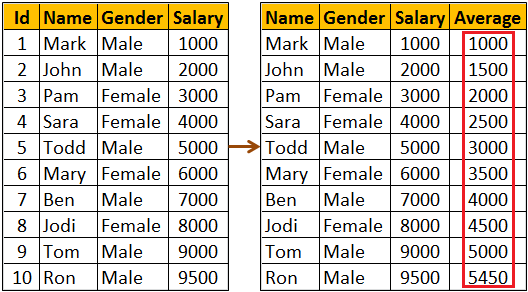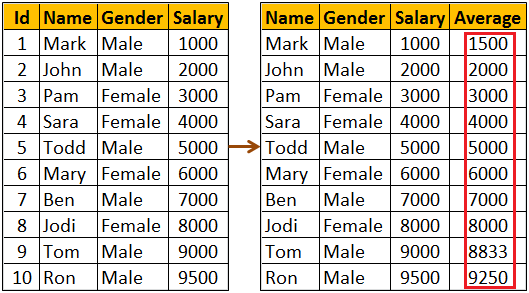HI WELCOME TO SIRING

# Window functions in SQL Server

### we will discusswindow functions in SQL Server

In SQL Server we have different categories of window functions
• Aggregate functions - AVG, SUM, COUNT, MIN, MAX etc..
• Ranking functions - RANK, DENSE_RANK, ROW_NUMBER etc..
• Analytic functions - LEAD, LAG, FIRST_VALUE, LAST_VALUE etc...
OVER Clause defines the partitioning and ordering of a rows (i.e a window) for the above functions to operate on. Hence these functions are called window functions. The OVER clause accepts the following three arguments to define a window for these functions to operate on.
• ORDER BY : Defines the logical order of the rows
• PARTITION BY : Divides the query result set into partitions. The window function is applied to each partition separately.
• ROWSor RANGE clause : Further limits the rows within the partition by specifying start and end points within the partition.
The default for ROWS or RANGE clause is
RANGE BETWEEN UNBOUNDED PRECEDING AND CURRENT ROW

Let us understand the use of ROWS or RANGE clause with an example.

Compute average salary and display it against every employee row as shown below.We might think the following query would do the job.
SELECT Name, Gender, Salary,
AVG(Salary) OVER(ORDER BY Salary) AS Average
FROM Employees

As you can see from the result below, the above query does not produce the overall salary average. It produces the average of the current row and the rows preceeding the current row. This is because, the default value of ROWS or RANGE clause (RANGE BETWEEN UNBOUNDED PRECEDING AND CURRENT ROW) is applied.To fix this, provide an explicit value for ROWS or RANGE clause as shown below. ROWS BETWEEN UNBOUNDED PRECEDING AND UNBOUNDED FOLLOWING tells the window function to operate on the set of rows starting from the first row in the partition to the last row in the partition.

SELECT Name, Gender, Salary,
AVG(Salary) OVER(ORDER BY Salary ROWS BETWEEN
UNBOUNDED PRECEDING AND UNBOUNDED FOLLOWING) AS Average
FROM EmployeesThe same result can also be achieved by using RANGE BETWEEN UNBOUNDED PRECEDING AND UNBOUNDED FOLLOWING

What is the difference between ROWS and RANGE
We will discuss this in a later video

The following query can be used if you want to compute the average salary of
1. The current row
2. One row PRECEDING the current row and
3. One row FOLLOWING the current row

SELECT Name, Gender, Salary,
AVG(Salary) OVER(ORDER BY Salary
ROWS BETWEEN 1 PRECEDING AND 1 FOLLOWING) AS Average
FROM Employees